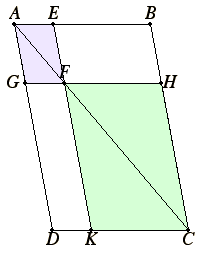# Proposition 24

In any parallelogram the parallelograms about the diameter are similar both to the whole and to one another.

Let ABCD be a parallelogram, and AC its diameter, and let EG and HK be parallelograms about AC.

I say that each of the parallelograms EG and HK is similar both to the whole ABCD and to the other.VI.2

For, since EF is parallel to a side BC of the triangle ABC, proportionally, BE is to EA as CF is to FA.

VI.2

Again, since FG is parallel to a side CD of the triangle ACD, proportionally, CF is to FA as DG is to GA.

But it was proved that CF is to FA as BE is to EA, therefore BE is to EA as DG is to GA. Therefore, taken jointly, BA is to AE as DA is to AG, and, alternately, BA is to AD as EA is to AG.

Therefore in the parallelograms ABCD and EG, the sides about the common angle BAD are proportional.

I.29

And, since GF is parallel to DC, the angle AFG equals the angle ACD, and the angle DAC is common to the two triangles ADC and AGF, therefore the triangle ADC is equiangular with the triangle AGF.

For the same reason the triangle ACB is also equiangular with the triangle AFE, and the whole parallelogram ABCD is equiangular with the parallelogram EG.

Therefore, proportionally, AD is to DC as AG is to GF, DC is to CA as GF is to FA, AC is to CB as AF is to FE, and CB is to BA as FE is to EA.

V.22

And, since it was proved that DC is to CA as GF is to FA, and AC is to CB as AF is to FE, therefore, ex aequali, DC is to CB as GF is to FE.

VI.Def.1

Therefore in the parallelograms ABCD and EG the sides about the equal angles are proportional. Therefore the parallelogram ABCD is similar to the parallelogram EG.

For the same reason the parallelogram ABCD is also similar to the parallelogram KH. Therefore each of the parallelograms EG and HK is similar to ABCD.

VI.21

But figures similar to the same rectilinear figure are also similar to one another, therefore the parallelogram EG is also similar to the parallelogram HK.

Therefore, in any parallelogram the parallelograms about the diameter are similar both to the whole and to one another.

Q.E.D.

## Guide

With this proposition Euclid returns to applications of areas. Back in proposition I.45 rectilinear areas were applied to lines. In the upcoming propositions VI.28 and VI.29, rectilinear areas will be applied to lines but the areas will fall short of or extend beyond the end of the lines. Those propositions geometric solve two kinds of quadratic equations. This proposition is preparatory to them. It is used in the proofs of VI.26 (its converse) and VI.29.